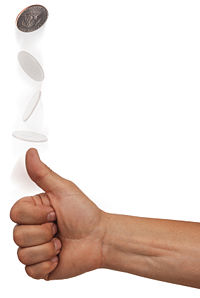# Coin toss betting having no loss no win puzzle

939.4K Views

Two friends were betting. One said to the other, “The coin will be flipped twenty times and each time the coin lands on head, I will give you \$2 and each time it lands on tale, you will give me \$3.” After flipping the coin for twenty times not a single penny was exchanged among them.

How many times did the coin land on heads ?12 Heads and 8 Tails => 12 x 2 = 24 counter-balanced by 8 x 3 = 24.

Solution: Assume that the coin landed ‘X’ times heads. Since the coin was tossed 20 times, the coin must have landed tails-up  20 – X times.
Since each ‘Heads’ costs the first person \$2, the total due = 2X
whereas
his friend would lose \$3 for every ‘tails’, adding up to (20 – X) x 3 = 60 – 3X

Since no money was exchanged,

2X = 60 – 3X

=> 5X = 60 => X = 12. Hence the result,

Let us suppose no of head occurences be x and number of tail ocurrennces will be 20-x ;since total no of throws is20,
now according to question it is given that for each head  one of the freinds gains \$2 so total gain is 2*x.Similarly total loss is 3*(20-x).
also 2*x=3*(20-x)8
From the equation x=12.
Therefore number of heads is 12 and number of tails is 8